homechevron_rightStudychevron_rightMath

# Volume of geometric shapes

Calculates volume of geometric shapes: cube, prism, pyramid, frustum, cone, cylinder, sphere, ellipsoid.

### This page exists due to the efforts of the following people:

#### Timur

This article contains a collection of calculators for calculating geometric figures' volume. Calculation formulas primary source: Spiegel, Murray R. Mathematical Handbook of Formulas and Tables. Schaum's Outline Series in Mathematics. McGraw-Hill Book Co., 1968.

## Volume of a cube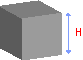Cube dimensions

Formula:#### Volume of a cube

Side length
Digits after the decimal point: 5
Volume

## Volume of a rectangular prism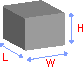Rectangular prism dimensions

Formula:#### Volume of a rectangular prism

Digits after the decimal point: 5
Volume

## Volume of a pyramid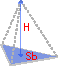Pyramid dimensions

Formula:#### Volume of a pyramid

Area of a pyramid base.
Digits after the decimal point: 5
Volume

## Volume of a frustum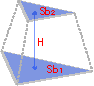Frustum dimensions

Formula:#### Volume of a frustum

Digits after the decimal point: 5
Volume

## Volume of a cone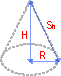Cone dimensions

Formula:#### Volume of a cone

Digits after the decimal point: 5
Volume

## Volume of a cylinder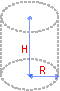Cylinder dimensions

Formula:#### Volume of a cylinder

Digits after the decimal point: 5
Volume

## Volume of a sphere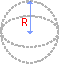Sphere dimensions

Formula:#### Volume of a sphere

Digits after the decimal point: 5
Volume

## Volume of a ellipsoid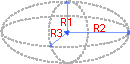Ellipsoid dimensions

Formula:#### Volume of an ellipsoid

Digits after the decimal point: 5
Volume

## Volume of a torus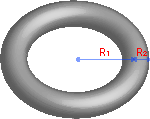Torus dimensions

Formula:#### Volume of a torus

Digits after the decimal point: 5
Volume

URL copied to clipboard

#### Similar calculatorsPLANETCALC, Volume of geometric shapes